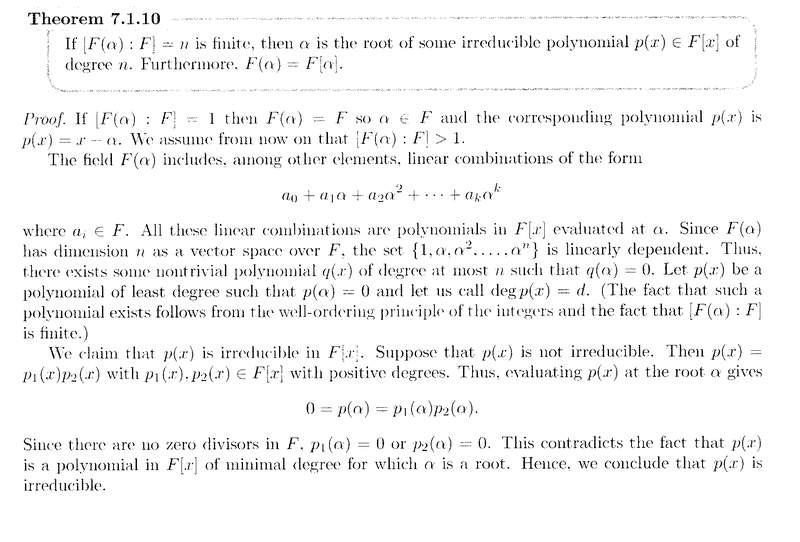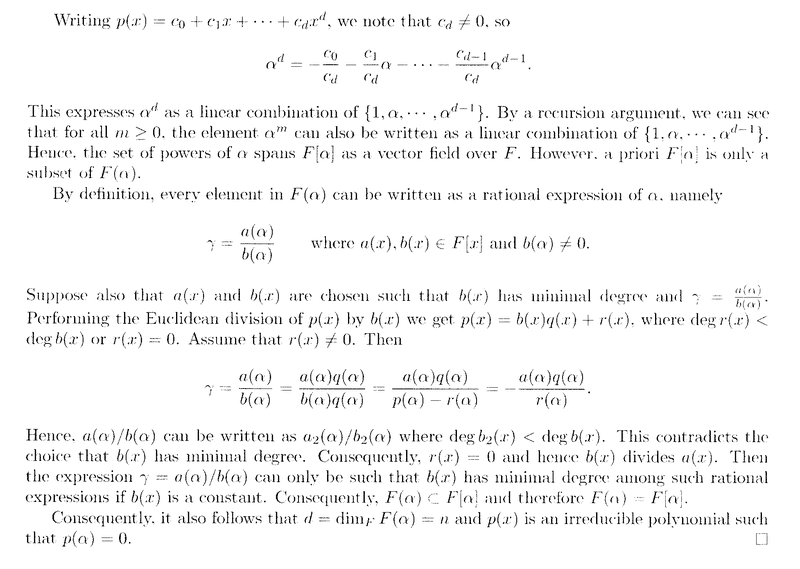# Field Extensions - Lovett, Theorem 7.1.10 - Another question

• I
• Math Amateur

#### Math Amateur

Gold Member
MHB
I am reading "Abstract Algebra: Structures and Applications" by Stephen Lovett ...

I am currently focused on Chapter 7: Field Extensions ... ...

I need help with another aspect of the proof of Theorem 7.1.10 ...

Theorem 7.1.10, and its proof, reads as follows:In the proof of the above Theorem, towards the end of the proof, Lovett concerns himself with proving that ##F( \alpha ) \subset F[ \alpha ]## ... ...

To do this he points out that every element in ##F( \alpha )## can be written as a rational expression of ##\alpha##, namely ...

##\gamma = \frac{ a( \alpha )}{ b( \alpha )}##

where ##a( \alpha ), b( \alpha ) \in F[x]## and ##b( \alpha ) \neq 0## ...

Lovett then says ...

" ... ... Suppose also that ##a( \alpha )## and ##b( \alpha )## are chosen such that ##b( \alpha )## has minimal degree and ##\gamma = \frac{ a( \alpha )}{ b( \alpha )}##. ... ... "

What does Lovett mean by choosing ##a( \alpha )## and ##b( \alpha )## such that ##b( \alpha )## has minimal degree ... ... ?

It cannot mean choosing special elements for ##b( \alpha )## ... as then ##\gamma## would not be a representative element of ##F( \alpha )## ...

Can someone please clarify this issue ...

Peter

EDIT Does it just mean that when we have ... for example ...

##\gamma = \frac{ a( \alpha )}{ b( \alpha )} = \frac{ x^3 - 3x }{ x^4 + 7x }##

we just (in this case, for example) we just 'cancel' the ##x## ... and similarly for other examples ...

#### Attachments

Yes.

The representation of a number ##\gamma## as a quotient ##\frac{a(\alpha)}{b(\alpha)}## is usually not unique.
However, the set ##\mathcal{Q}_\gamma = \left\{ \left. \frac{a(x)}{b(x)} \,\right\vert \,a,b \in F[x]\, , \,\gamma = \frac{a(\alpha)}{b(\alpha)} \right\} ## can be partially ordered by the degree of ##b(x)##. Furthermore, ##\deg b(x) \geq 0##, and thus there must be a minimal element in any ordered chain in ##\mathcal{Q}_\gamma##. Such an element is chosen.

This doesn't mean this element is unique, as we still can have common factors of nominator and denominator of degree ##0##, i.e. elements of ##F##, or even different polynomials ##b(x)## and ##b'(x)## in the representation of ##\gamma##. But as the argument only relies on the degree of ##b(x)##, we don't care, whether elements of ##F## have been canceled or not, or which ##b(x)## of minimal degree (i.e. which ordered chain in ##\mathbb{Q}_\gamma##\,) we chose. Even ##\alpha## itself can be in ##F##, in which case nothing would have been to prove, so we can rule out this case, too, if we like.

Yes.

The representation of a number ##\gamma## as a quotient ##\frac{a(\alpha)}{b(\alpha)}## is usually not unique.
However, the set ##\mathcal{Q}_\gamma = \left\{ \left. \frac{a(x)}{b(x)} \,\right\vert \,a,b \in F[x]\, , \,\gamma = \frac{a(\alpha)}{b(\alpha)} \right\} ## can be partially ordered by the degree of ##b(x)##. Furthermore, ##\deg b(x) \geq 0##, and thus there must be a minimal element in any ordered chain in ##\mathcal{Q}_\gamma##. Such an element is chosen.

This doesn't mean this element is unique, as we still can have common factors of nominator and denominator of degree ##0##, i.e. elements of ##F##, or even different polynomials ##b(x)## and ##b'(x)## in the representation of ##\gamma##. But as the argument only relies on the degree of ##b(x)##, we don't care, whether elements of ##F## have been canceled or not, or which ##b(x)## of minimal degree (i.e. which ordered chain in ##\mathbb{Q}_\gamma##\,) we chose. Even ##\alpha## itself can be in ##F##, in which case nothing would have been to prove, so we can rule out this case, too, if we like.

Thanks fresh_42 ... most helpful ...

BUT ... just a couple more clarifications pertaining to Lovett's argument that ##b(x)## must be a constant (an argument I am having trouble fully understanding ... )

(QUESTION 1) Lovett brings the minimal polynomial ##p(x)## into the argument ... why is he doing this ... what is his objective in this matter ... ?

Further ... ... Lovett writes ... :

" ... ... Hence ##a( \alpha ) / b( \alpha )## can be written as ##a_2( \alpha ) / b_2( \alpha )## where ##\text{ deg } b_2( x ) \lt \text{ deg } b( x)##. This contradicts the choice that ##b( x)## has minimal degree. Consequently, ##r(x) = 0## and hence ##b(x)## divides ##a(x)##. ... .. "

(QUESTION 2) I cannot see how in this argument Lovett concludes that ##b(x)## divides ##a(x)## ... ... ? Can you help ...?

Then Lovett writes:

" ... ... Then the expression ##\gamma = a( \alpha ) / b( \alpha )## can only be such that ##b(x)## has minimal degree among such rational expressions if ##b(x)## is a constant. ... ... "

(QUESTION 3) I do not follow this argument that ##b(x)## must be a constant ... can you help ... ?

Peter

Last edited:
Hi Peter,
(QUESTION 1) Lovett brings the minimal polynomial ##p(x)## into the argument ... why is he doing this ... what is his objective in this matter ... ?
About the "why" question: (I'll try not to write "Because it works." and guess a motivation instead. Truth is, finding a proof is usually a process of trial and error. You still can see this in Lovett's style, which mostly is a sequence of many small contradiction arguments. Not very pleasant to read.)

If we gather all we have about ##\gamma ## and ##\alpha ##, then it is ##\gamma = \frac{a(\alpha)}{b(\alpha)}## and ##p(\alpha)=0##, so it makes sense to relate those three polynomials ( ##a,b,p## ), i.e. see what divisions bring. The goal is to show ##b(x)=b_0 \in F## so that all quotients ## \gamma ## are already in ##F[\alpha]##. This means the degree of ##b(x)## has to be ##1## for this purpose. Division is a good method in algebra to reduce degrees or similar valuations or to use it in an induction argument.
Further ... ... Lovett writes ... :

" ... ... Hence ##a( \alpha ) / b( \alpha )## can be written as ##a_2( \alpha ) / b_2( \alpha )## where ##\text{ deg } b_2( x ) \lt \text{ deg } b( x)##. This contradicts the choice that ##b( x)## has minimal degree. Consequently, ##r(x) = 0## and hence ##b(x)## divides ##a(x)##. ... .. "
##a_2(\alpha) = a(\alpha)\cdot q(\alpha)## and ##b_2(\alpha)=-r(\alpha)##. We also have (Euclid's algorithm) ##\deg b_2(x) = \deg r(x) < \deg b(x)##. Minimality of ##\deg b(x)## in the representation of ##\gamma ##, however, implies that ##b_2(x)=r(x)=0##. (We have chosen ##b(x)## this way, see my earlier post.)

Now I'm also lost. I assume it's a typo, since ##r(x)=0## implies ##b(x) \mid p(x)##. Now ##p(x)## is irreducible, and therefore a factor ##b(x) ## of it has to be a unit, i.e. an element of ##F\, : \, b(x)=b_0 \in F##.

(QUESTION 2) I cannot see how in this argument Lovett concludes that ##b(x)## divides ##a(x)## ... ... ? Can you help ...?
Me neither, see above. ##a(x)## should be replaced by ##p(x)## here.
Then Lovett writes:

" ... ... Then the expression ##\gamma = a( \alpha ) / b( \alpha )## can only be such that ##b(x)## has minimal degree ##b(x)## has minimal degree among such rational expressions if ##b(x)## is a constant. ... ... "

(QUESTION 3) I do not follow this argument that ##b(x)## must be a constant ... can you help ... ?
We have written ##\gamma = \frac{a_2(\alpha)}{b_2(\alpha)}## with the settings for ##a_2(x),b_2(x)## I mentioned above. But ##\deg b(x)## was chosen minimal among all possible representations of ##\gamma## in this way.

I hope my answer on your first question covers the other two.

I've forgotten one point. The argument that ##b(x)## has to be a unit uses the fact, that otherwise ##q(x)=q_0## would be the unit (by irreducibility of ##p(x)##). But then ##0=p(\alpha)=b(\alpha)\cdot q(\alpha) = b(\alpha) \cdot q_0## and ##b(\alpha)=0## which can't be by the choice of ##b(\alpha)## as the denominator of ##\gamma##. Thus ##b(x)## has to be the unit.

Last edited:
•Math Amateur
Hi Peter,

About the "why" question: (I'll try not to write "Because it works." and guess a motivation instead. Truth is, finding a proof is usually a process of trial and error. You still can see this in Lovett's style, which mostly is a sequence of many small contradiction arguments. Not very pleasant to read.)

If we gather all we have about ##\gamma ## and ##\alpha ##, then it is ##\gamma = \frac{a(\alpha)}{b(\alpha)}## and ##p(\alpha)=0##, so it makes sense to relate those three polynomials ( ##a,b,p## ), i.e. see what divisions bring. The goal is to show ##b(x)=b_0 \in F## so that all quotients ## \gamma ## are already in ##F[\alpha]##. This means the degree of ##b(x)## has to be ##1## for this purpose. Division is a good method in algebra to reduce degrees or similar valuations or to use it in an induction argument.

##a_2(\alpha) = a(\alpha)\cdot q(\alpha)## and ##b_2(\alpha)=-r(\alpha)##. We also have (Euclid's algorithm) ##\deg b_2(x) = \deg r(x) < \deg b(x)##. Minimality of ##\deg b(x)## in the representation of ##\gamma ##, however, implies that ##b_2(x)=r(x)=0##. (We have chosen ##b(x)## this way, see my earlier post.)

Now I'm also lost. I assume it's a typo, since ##r(x)=0## implies ##b(x) \mid p(x)##. Now ##p(x)## is irreducible, and therefore a factor ##b(x) ## of it has to be a unit, i.e. an element of ##F\, : \, b(x)=b_0 \in F##.

Me neither, see above. ##a(x)## should be replaced by ##p(x)## here.

We have written ##\gamma = \frac{a_2(\alpha)}{b_2(\alpha)}## with the settings for ##a_2(x),b_2(x)## I mentioned above. But ##\deg b(x)## was chosen minimal among all possible representations of ##\gamma## in this way.

I hope my answer on your first question covers the other two.

I've forgotten one point. The argument that ##b(x)## has to be a unit uses the fact, that otherwise ##q(x)=q_0## would be the unit (by irreducibility of ##p(x)##). But then ##0=p(\alpha)=b(\alpha)\cdot q(\alpha) = b(\alpha) \cdot q_0## and ##b(\alpha)=0## which can't be by the choice of ##b(\alpha)## as the denominator of ##\gamma##. Thus ##b(x)## has to be the unit.

Thanks fresh_42 ... just reflecting on your post ...

But one quick clarification ...

You write:

" ... ... The goal is to show ##b(x)=b_0 \in F## so that all quotients ## \gamma ## are already in ##F[\alpha]##. This means the degree of ##b(x)## has to be ##1## for this purpose. ... ... "

Can you explain your reasoning that the degree of ##b(x)## has to be ##1## ... ...

If ##b(x)=b_0 \in F## then doesn't the degree of ##b(x)## have to be ##0## ... ... ?

Hope you can clarify ... ... must be misunderstanding you somehow ...

Peter

Yep, it's late up here ... Of course ##0##, sorry.

•Math Amateur
Yep, it's late up here ... Of course ##0##, sorry.

•